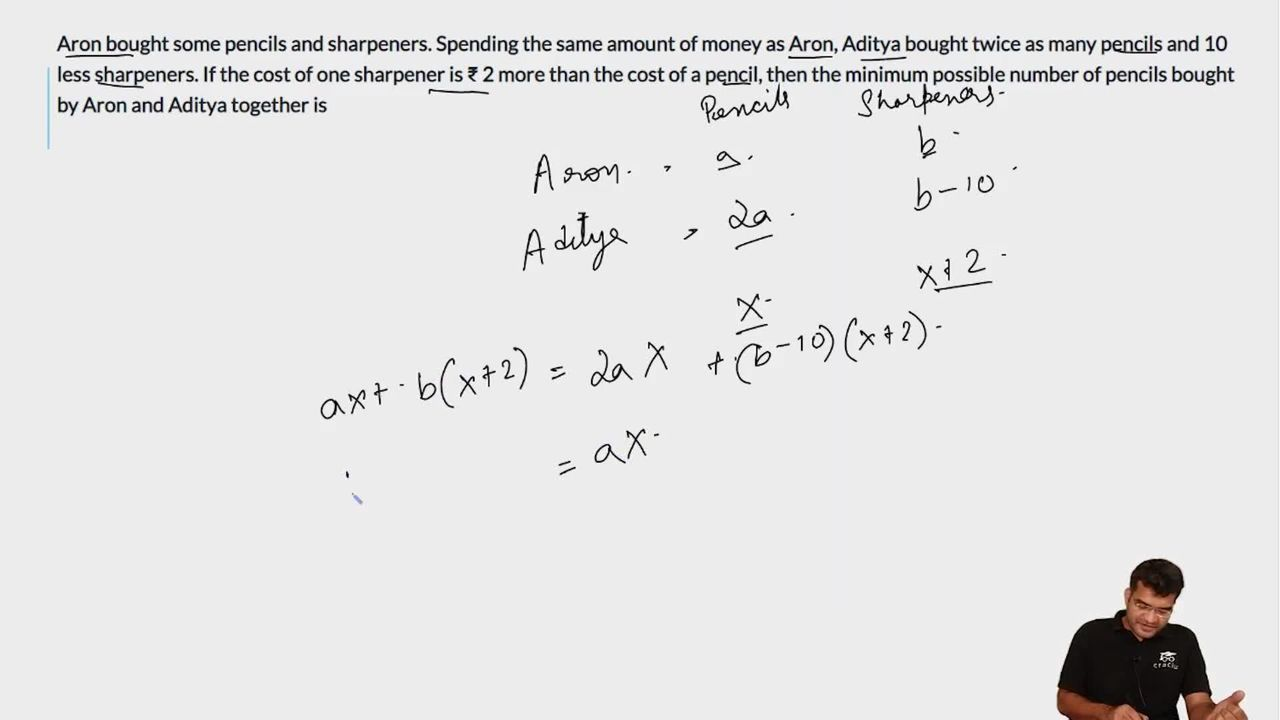Question 73

# Aron bought some pencils and sharpeners. Spending the same amount of money as Aron, Aditya bought twice as many pencils and 10 less sharpeners. If the cost of one sharpener is ₹ 2 more than the cost of a pencil, then the minimum possible number of pencils bought by Aron and Aditya together is

Solution

Let the number of pencils bought by Aron be "p" and the cost of each pencil be "a".

Let the number of sharpeners bought Aron be "s" and the cost of each sharpener be "b".

Now amount spent by Aron will be (pa)+(sb)

Aditya bought (2p) pencils and (s-10) sharpeners. Amount spent will be (2pa)+(s-10)b

Amount spent in both the cases is same

pa + sb = 2pa + (s-10)b or pa=10b

Now its given in the question that cost of sharpener is 2 more than pencil i.e. b=a+2

pa= 10a+20 or a=20/(p-10)

Now the number of pencils has to be minimum, for that we have to find smallest "p" such that both "p" and "a" are integers. The smallest such value is p=11 . Total number of pencils bought will be p+2p=11+22=33

### View Video Solution